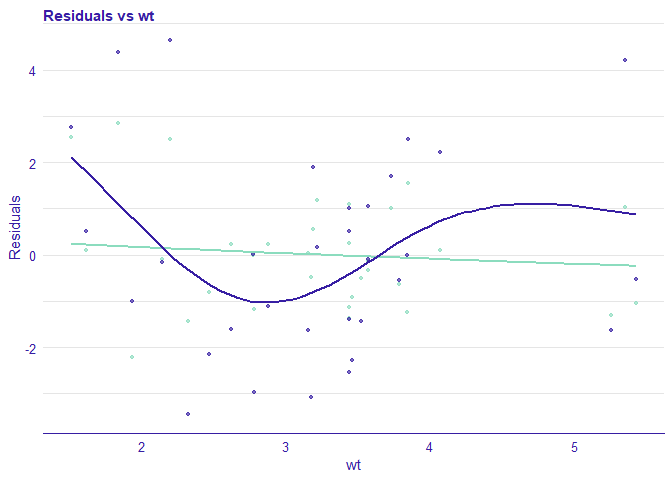## Overview

Package auditor is a tool for model-agnostic validation. Implemented techniques facilitate assessing and comparing the goodness of fit and performance of models. In addition, they may be used for the analysis of the similarity of residuals and for the identification of outliers and influential observations. The examination is carried out by diagnostic scores and visual verification. Due to the flexible and consistent grammar, it is simple to validate models of any classes.

auditor is a part of DrWhy collection of tools for Visual Exploration, Explanation and Debugging of Predictive Models.

auditor’s pipeline: model %>% DALEX::explain() %>% plot(type=…)

## Installation

Stable version from CRAN:

install.packages("auditor")

Developer version from GitHub:

source("https://install-github.me/ModelOriented/auditor")

# or with the devtools package
devtools::install_github("ModelOriented/auditor")

## Demo

Run the code below or try the auditor.

library(auditor)
library(randomForest)
data(mtcars)

# fitting models
model_lm <- lm(mpg ~ ., data = mtcars)
set.seed(123)
model_rf <- randomForest(mpg ~ ., data = mtcars)

# creating objects with 'explain' function from the package DALEX
# that contains all necessary components required for further processing
exp_lm <- DALEX::explain(model_lm, data = mtcars, y = mtcars$mpg, verbose = FALSE) exp_rf <- DALEX::explain(model_rf, data = mtcars, y = mtcars$mpg, label = "rf", verbose = FALSE)

# create explanation  objects
mr_lm <- model_residual(exp_lm)
mr_rf <- model_residual(exp_rf)

# generating plots
plot_residual(mr_lm, mr_rf, variable = "wt", smooth = TRUE)## Short overview of plots

Column type contains character that should be passed to parameter type= when using plot() function. Regr and Class columns indicate whether plot can be used for regression and classification models.

Name of a plot Function Interactive version Type Regr Class
Autocorrelation Function plot_acf() plotD3_acf() “acf” yes yes
Autocorrelation plot_autocorrelation() plotD3_autocorrelation() “autocorrelation” yes yes
Influence of Observations plot_cooksdistance() plotD3_cooksdistance() “cooksdistance” yes yes
Half-Normal plot_halfnormal() plotD3_halfnormal() “halfnormal” yes yes
LIFT Chart plot_lift() plotD3_lift() “lift” no yes
Model Correlation plot_correlation() - “correlation” yes yes
Principal Component Analysis of Models plot_pca() - “pca” yes yes
Work on this package was financially supported by the NCN Opus grant 2016/21/B/ST6/02176.